Tamilnadu State Board New Syllabus Samacheer Kalvi 4th Science Guide Pdf Term 1 Chapter 3 Work and Energy Text Book Back Questions and Answers, Notes.

## Tamilnadu Samacheer Kalvi 4th Science Solutions Term 1 Chapter 3 Work and Energy

### Samacheer Kalvi 4th Science Guide Work and Energy Text Book Back Questions and AnswersI. Use the correct word to fill the blanks :

(Ramp, Simple machines, Work, Energy, Pulley)

1. ___________ is said to be done when a force is acting on it.
Work

Question 2.
The ability to do work is __________.
Energy

Question 3.
___________ is a machine made up of wheel and rope.
Pulley

Question 4.
____________ help us to make work easier.
Simple machines

Question 5.
An example for inclined plane is ____________.
RampII. Rearrange the letters and find out the names of the tools :

Question 1.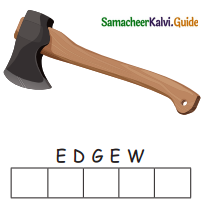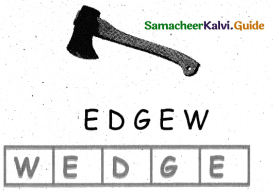Question 2.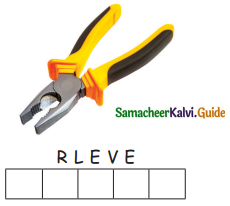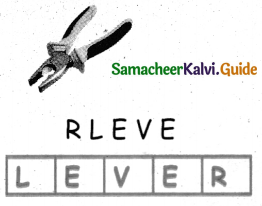Question 3.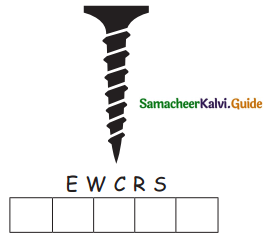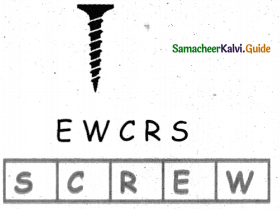III. Match the following :

Question 1.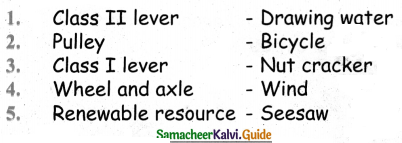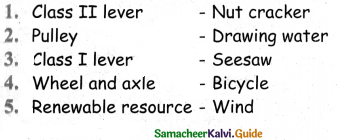IV. Classify the things below : (Text Book Page No. 109)

Question 1.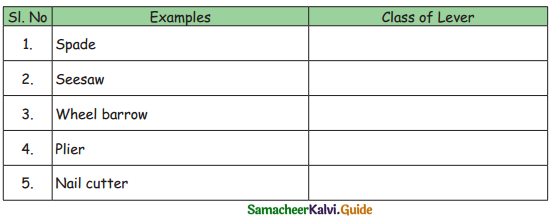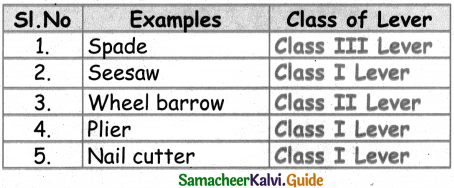Question 1.
What is the unit of energy?
The S.I. Unit of energy is the joule.

Question 2.
Name some simple machines.
Simple machines are pulley, wedge, inclined plane, screw, lever, wheel and axle.

Question 3.
What is a first order lever?
When the fulcrum is between the effort and the load, it is known as Class I lever.
Example: scissors, pliers, seesaw.

Question 4.
Lemon juicer belongs to which type of lever? Why?
Lemon juicer belongs to Class II Lever. In a lemon squeezer, the lemon (load) is at the middle, the load is at the center of effort and fulcrum.

Question 5.
Define work.
An action in which one exerts a force to move an object is known as work.

Question 6.
Write any three types of energy.

• Mechanical energy.
• Thermal energy.
• Chemical energy.### Samacheer Kalvi 4th Science Guide Work and Energy In Text Questions and Answers

Try to Answer (Text Book Page No. 104)

Observe the picture and put a tick ✓) if work is done and put a cross (x) if work is not done.
Question 1.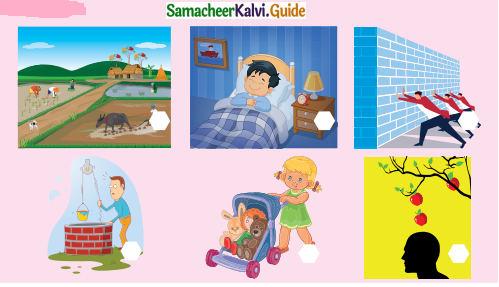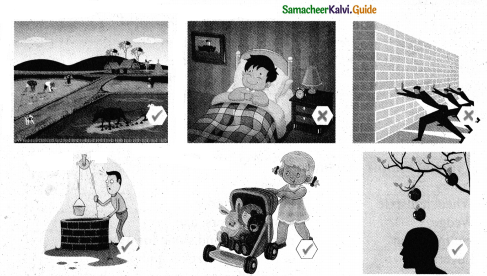Let us do (Text Book Page No. 104)

Mention whether work is done or not in the following activity.
Question 1.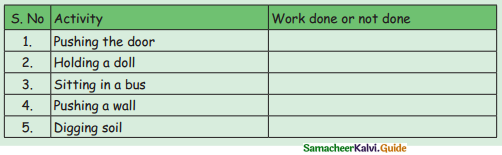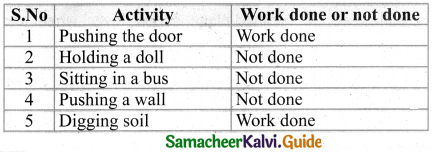Try to Answer (Text Book Page No. 109)

Identify and mention the types of simple machines.
Question 1.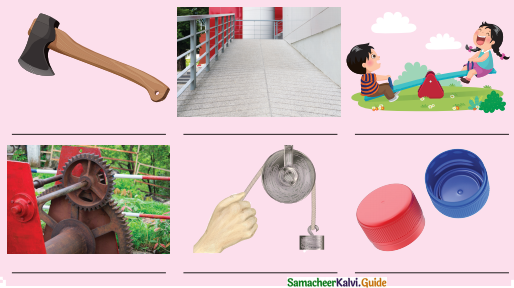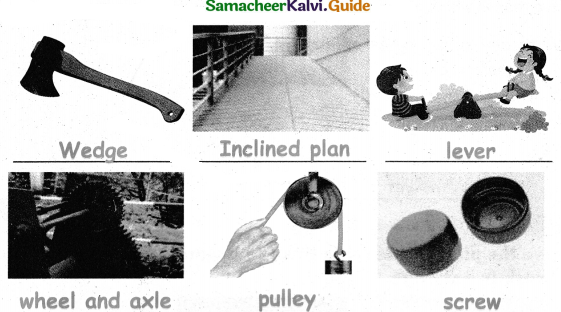Let us do (Text Book Page No. 109)

Complete the table.
Question 1.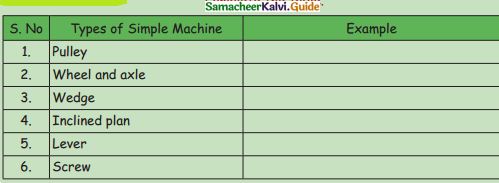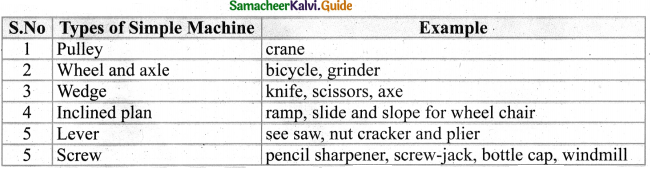Try to Answer (Text Book Page No. 111)

Name the load, effort and fulcrum.
Question 1.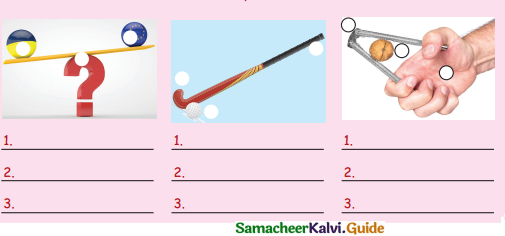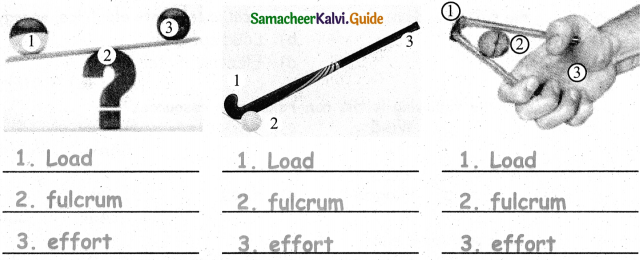### Samacheer Kalvi 4th Science Guide Work and Energy Additional Questions and AnswersI. Pick out the odd one :

Question 1.
Mechanical energy, Heat energy, Chemical energy, Food
Food

Question 2.
Scissors, broomstick, wrench, sea saw
Broomstick

Question 3.
petrol, coal, natural gas, water.
WaterII. Say true or false :

Question 1.
Chemical energy is one of the types of lever.
False

Question 2.
Simple machines usually exchange a larger force to move a heavy object.
False

Question 3.
The idea of a simple machine originated with the Greek philosopher Archimedes.
True

Question 4.
A lever is used to multiply the force.
TrueIII. Match the following :

Question 1.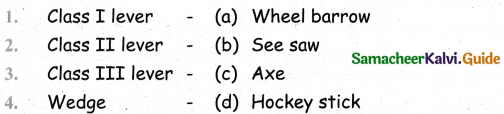1. – (b)
2. – (a)
3. – (d)
4. – (c)IV. Choose the correct answer :

Question 1.
second order lever ___________.
(a) Scissors
(b) lemon squeezer
(c) Tongs
(b) lemon squeezer

Question 2.
Which of the following device converts chemical energy into electrical energy?
(a) Battery
(b) Loud speaker
(c) Solar cell
(d) Electric motor
(q) Battery

Question 3.
Which among the following is the non-renewable resources?
(a) Sun
(b) Wind
(c) Water
(d) Petrol
(d) Petrol

Question 4.
SI unit of energy is ______________.
(a) Joule
(b) kilogram
(c) Mass
(d) Watt
(a) Joule

Question 5.
A is a push or a pull
(a)Force
(b)Friction
(c) Simple machine
(d) pulley
(b) Friction

Question 6.
Simple machines makes work easier by trading _____________ for force.
(a) Motion
(b) Work
(c) Friction
(d) Distance
(b) Work

Question 7.
A force can cause an object to change its
(a) Shape
(b) Speed
(c) Direction
(d) All the above
(d) All the aboveV. Fill in the blanks :

Question 1.
A ___________ should act on an object.
Water

Question 2.
A pulley is a machine made up of a _____________.
Wheel

Question 3.
_____________ is used to split materials.
Wedge

Question 4.
The screw is used to raise _____________.
Weights

Question 5.
The _____________ and sharpener are held together by screw.VI. Answer the following questions in one or two sentences :

Question 1.
What is non-renewable resources?
The resources which are not easily replaced once used are called the non renewable resources.
Example: Petrol, Coal and Natural gas.

Question 2.

• Renewable sources of energy are replaced naturally over a period of time.
• We can keep using these sources for a long period of time. Since the beginning of human life, we have been using these resources.
• We use these resources for light, transport, cooking, heating. Eg: Sun, Wind and Water.

Question 3.
Write the two main conditions are needed for work to be done?
Two main conditions are needed for work to be done.

1. A force should act on an object.
2. Object should move from one place to another.

Question 4.
What is work?
An action in which one exerts a force to move an object is known as work.

Question 5.
Define energy?

• Energy is defined as capacity for doing work.
• Energy must be transferred to an object in order to do work.

Question 6.
Write the type of energy.

• Mechanical energy.
• Thermal energy
• Chemical energy
• Electrical energy
• Non-renewable energy
• Renewable energyQuestion 7.
What is simple machine?